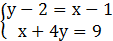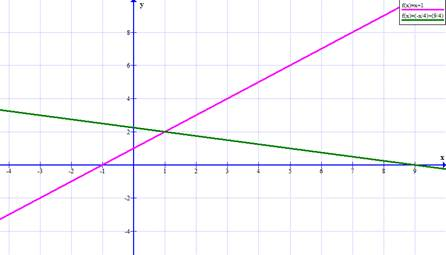### Sample Problem

Solve the system graphically:Round your answers to the nearest whole number:

x=

y=

#### Solutiony=x+1

4y=9-x , y=(9-x)/4=(-x/4)+(9/4)

Put these two equations into your calculator and find the intersection point.x=1 ; y=2